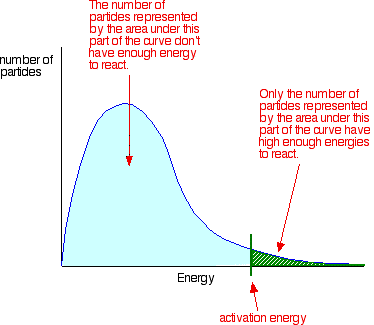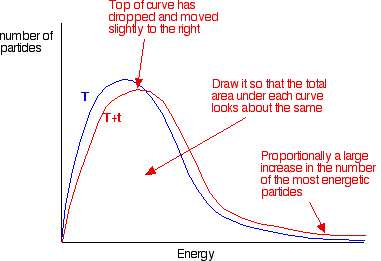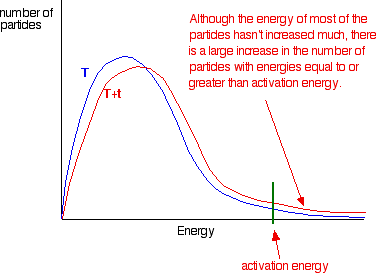Need help? Email help@shout.education

# The Effect of Temperature on Rates of Reaction

This page describes and explains the way that changing the temperature affects the rate of a reaction. It assumes that you are already familiar with basic ideas about the collision theory, and with the Maxwell-Boltzmann distribution of molecular energies in a gas.

Note: If you haven't already read the page about collision theory, you should do so before you go on.

## The Facts

### What Happens?

As you increase the temperature the rate of reaction increases. As a rough approximation, for many reactions happening at around room temperature, the rate of reaction doubles for every 10°C rise in temperature.

You have to be careful not to take this too literally. It doesn't apply to all reactions. Even where it is approximately true, it may be that the rate doubles every 9°C or 11°C or whatever. The number of degrees needed to double the rate will also change gradually as the temperature increases.

Note: You will find the effect of temperature on rate explored in a slightly more mathematical way on a separate page.

### Examples

Some reactions are virtually instantaneous – for example, a precipitation reaction involving the coming together of ions in solution to make an insoluble solid, or the reaction between hydrogen ions from an acid and hydroxide ions from an alkali in solution. So heating one of these won't make any noticeable difference to the rate of the reaction.

Almost any other reaction you care to name will happen faster if you heat it – either in the lab, or in industry.

## The Explanation

### Increasing the Collision Frequency

Particles can only react when they collide. If you heat a substance, the particles move faster and so collide more frequently. That will speed up the rate of reaction.

That seems a fairly straightforward explanation until you look at the numbers!

It turns out that the frequency of two-particle collisions in gases is proportional to the square root of the kelvin temperature. If you increase the temperature from 293 K to 303 K (20°C to 30°C), you will increase the collision frequency by a factor of:

\sqrt{\frac{303}{293}} = 1.017

That's an increase of 1.7% for a 10° rise. The rate of reaction will probably have doubled for that increase in temperature – in other words, an increase of about 100%. The effect of increasing collision frequency on the rate of the reaction is very minor. The important effect is quite different

### The Key Importance of Activation Energy

Collisions only result in a reaction if the particles collide with enough energy to get the reaction started. This minimum energy required is called the activation energy for the reaction.

Note: What follows assumes you have a reasonable idea about activation energy and its relationship with the Maxwell-Boltzmann distribution. This is covered on the introductory page about collision theory.

You can mark the position of activation energy on a Maxwell-Boltzmann distribution to get a diagram like this:Only those particles represented by the area to the right of the activation energy will react when they collide. The great majority don't have enough energy, and will simply bounce apart.

To speed up the reaction, you need to increase the number of the very energetic particles – those with energies equal to or greater than the activation energy. Increasing the temperature has exactly that effect – it changes the shape of the graph.

In the next diagram, the graph labelled T is at the original temperature. The graph labelled T+t is at a higher temperature.If you now mark the position of the activation energy, you can see that although the curve hasn't moved very much overall, there has been such a large increase in the number of the very energetic particles that many more now collide with enough energy to react.Remember that the area under a curve gives a count of the number of particles. On the last diagram, the area under the higher temperature curve to the right of the activation energy looks to have at least doubled – therefore at least doubling the rate of the reaction.

### Summary

Increasing the temperature increases reaction rates because of the disproportionately large increase in the number of high energy collisions. It is only these collisions (possessing at least the activation energy for the reaction) which result in a reaction.